### Collaborative Choice

The effect is that the class all contribute to the free selection of a number which the teacher had predicted in advance.

To set up this trick you need to firstly choose the target number and write this down as your "prediction"! This should be done before the class have arrived for their lesson. Your prediction can be sealed in an envelope or, for more of a showy reveal, written on the back of a picture hanging in the room.

For the purposes of this explanation the target number is 36.9. Easy for you to remember as it is normal human body temperature (you knew that didn't you)?

So the trick begins with you asking a student at random to choose a number between 1 and 100 which you have written on the board like this: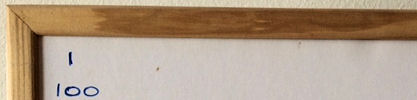If the student offers a number which is larger than the target number you replace the bottom number with the student's number, otherwise you replace the top number.

Let's imagine the student offers the number 40. Your board should now look like this:The next number you ask for has to be between 1 and 40 (the new top and bottom numbers).

Let's imagine the next five students you pick at random offer the numbers 20, 30, 35, 37.5 and 36.5. Your board should now look like this: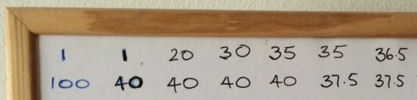Can you see how by deciding to put the students' numbers in either the top or bottom rows you are zooming in on the target number? Eventually one of the students will offer the target number. You then white it in large digits spanning both rows. You say something like "I think that's enough to ensure many of you have contributed to selecting the class' chosen number". You then reveal your prediction and all are amazed!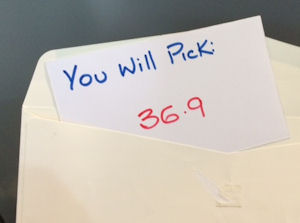## iPhone Calculation

This trick works best on the standard iPhone calculator though other calculators may have the same way of working and could also be used.

The effect is that pupils come up with random two and three digit numbers which you multiply together. Irrespective of what numbers they come up with the product will be the same as the number you had previously predicted.

To set up the trick you need to have previously chosen the large number as your prediction. This should be done before the class have arrived for their lesson. Your prediction can be sealed in an envelope or, for more of a showy reveal, written on the back of a picture hanging in the room.

The other essential part of the setup is to type your predicted number into the calculator then press the plus button, the zero button and the multiplication button … nothing else.

When the students call out their random two and three digit numbers you type them into the calculator pressing the multiplication button between each one. This should be done in full view of the students so that can see that you are not doing anything sneaky! After five or six numbers you press the equals sign and, as if by magic, the predicted number appears on the calculator screen. You then reveal your prediction and all are amazed!How does it work? Well it involves the principle of the Order of Operations or BIDMAS. Let's imagine the students' numbers were 19, 345, 21, 999 and 456 and your prediction was 1,601,863.

The numbers entered into the calculator were:

1601863 + 0 x 19 x 345 x 21 x 999 x 456

The students didn't see the first two numbers entered as you did this before they arrived. The calculator performs the multiplications before the addition so the product of zero is added to your prediction. Magic!

The effect is that you can add up ten numbers in your head faster than anyone in the class can do it with a calculator.

A volunteer from the class is chosen to be the calculator operator. Another student, with good handwriting, is chosen as the Scribe, to write on the board.

You, the teacher, and the calculator operator stand with your backs to the board so you can't see the numbers being written.

The scribe is told to write down a one digit number then, underneath that, to write a second one digit number.

A third number, the sum of the first and second numbers, should now be written down.

A fourth number, the sum of the second and third numbers, should also be written down.

The scribe continues to write down numbers, each one being the sum of the previous two, until there are ten numbers in the list altogether.

At this point you and the calculator operator turn to face the board in a race to find the total.

The trick is that rather than attempt to add all of the numbers together you simply multiply the seventh number by 11. It will give you the total that will take the calculator operator more time to work out. The class will be amazed at your numeracy skills!

How does it work? There are two tricks involved. The first is the quick way of multiplying by eleven and the other is related to the fact that this is a Fibonacci type sequence.

Let the first and second numbers selected be x and y respectively. The ten terms of the sequence will be x, y, x+y, x+2y, 2x+3y, 3x+5y, 5x+8y, 8x+13y, 13x+21y and 21x+34y.

The sum of this sequence is 55x + 88y which is 11 times 5x+8y.## Two Squares

This trick appears to be mathematical but perhaps it is not!

Can you change this square into two using only a pencil? You are not allowed to come anywhere near the screen (or whiteboard).The Trick: Carefully use the blunt end of a pencil to push up the lower eye lid of one eye and you will see double!

## Coming Together

Each student is asked to think of a number between 2 and 9 inclusive (or ace, jack, queen or king). Whatever number they have chosen, there are four cards in their pack with that value. Students are asked to shuffle the pack while thinking about their cards.Students are considered to have magical powers if two of their cards come together in the pack (well there might be one card between them but for the purposes of this exercise we will call that coming together).

Most students should find that this works for them ... so they have magic powers ... or have they?

The Trick: In fact this is an example where the odds are weighted in favour of a success ... can you estimate the probability from the results of this activity?

## Think of a number

• Multiply it by nine
• Subtract five
• If A=1, B=2, C=3 etc what letter is represented by the number you are thinking of now?
• Think of a country beginning with that letter
• Think of an animal beginning with the second letter of that country
• What is the colour of that animal?

"There aren't many grey elephants living in Denmark are there?"

Virtually everyone in the room will have thought of Denmark as their country and as elephant as their animal. Is this magic?

Students can now investigate the probability of this happening. Having first satisfied themselves that everyone will arrive at the letter D independently of their starting number, how many countries are there starting with D?

How many animals beginning with E? Of all the animals beginning with E how likely is a person to choose elephant? Perhaps a survey might answer this question.

Students could now be asked to make up for themselves a similar trick (but not using Elephants from Denmark). Try out the tricks on some other students and teachers.

Finally a demonstration of tree diagrams can be used to explain the probabilities of these tricks working.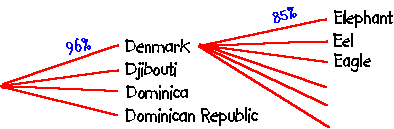Update November 2014: The 96% and 85% on the tree diagram above were made-up figures for illustrative purposes only. We have now conducted our own survey to see what those figures should be. You can see the results on the Predictive Survey Results page.]

You can amaze your students by pretending to add up a whole column of three digit numbers in a couple of seconds.

Ask three students to write three digits each on a piece of paper as if they are to be added and return the paper to you.

You write two more numbers below those. Choose your first number (which is the fourth one in the problem) so that the first number and fourth numbers add up to 999. Choose your next number so that it adds with the second number to give 999.

283
234
549
716
765

Give a student the paper and ask them to add up the numbers on a calculator. Then the student could return the paper to you. Look at it briefly as if you are adding the numbers in your head and announce the answer.

The answer will be 2000 plus the third number minus 2 which you could compute very quickly once the student returns the paper.

This could be followed by a class discussion about how this trick works ... students practise with friends.

## Letters In A Number

The Teacher writes a number on a large piece of paper. The students can see this going on but can't see which number it is. The paper is the put in an envelope or a box and put in a safe place in view of the students.

The Teacher then asks a student to think of a very large number. This number is written on the board in words.

"How many letters are in these words?" ... the answer is written underneath in words...."How many letters are in these words?" ... and so on repeating the process again and again till the list of numbers has reached the bottom of the board. The envelope is then opened to reveal the number FOUR which will be the last number written on the board.

F O U R

"What if we start with a different number?" ... another student volunteers a different starting number which eventually generates four again.

"Does this always happen?".. students try some of their own.

"Why does it always happen?"

Students now do an investigation looking at the properties of the number words in different languages. They can use foreign language dictionaries or the internet as appropriate. They should produce a write up of their findings for their folders.

## Mental Tricks

many calculations have short cuts or tricks associated with them which enable students to perform the calculations quickly in their heads.

A list of such tricks can be found here.

Here are a couple of examples:

Quick Square
Did you know that there is a quick way of squaring a two digit number which ends in 5?
Just multiply the first digit by that number plus one.... stick a 25 after your product and there's your answer .... simple ?

Example:

Q. What is 35 squared?
A. 3x4=12
.....now stick on the 25

Do you know why it works?

Times Eleven
The eleven times table has always been very easy to learn up to 9 x 11. Here's a way of multiplying large numbers by 11 too:

Example:

Q. What is 324 x 11 ?
A. Write down the first digit ... 3
.......Add the first and second digits ... 3 + 2 = 5
.......Add the second and third digits .. 2 + 4 = 6
.......Write down the last digit ........... 4

Try it yourself ... multiply 543 by 11

Do you know why it works?

Does it work for all three digit numbers?

## Cluedo

A large house plan made from 9 pieces of carpet (each 3m by 3m) is set out in the middle of a large room as shown:A student is dressed to look like the murderer(!) and stands on the carpet piece marked "Hall".

A teacher (acting as Sherlock Holmes) explains that the murderer will be tracked down in the house and arrested. Sherlock is blindfolded.

A second student throws the dice and states the number on the dice out loud. The murderer walks through that many doors on the plan of the house.

Sherlock says "I know the murderer is not in the kitchen (or name of other room) , so will someone please take away the kitchen"

A third student takes away the piece of carpet labelled "kitchen". the murder from now on cannot step on the area which was once the kitchen.

The process is repeated a number of times until Sherlock can say "I know that the murderer is in the ........... (name of room), you are under arrest.

"How does the trick work?"

Well if the murderer starts in the hall, four rooms are one door away (study, bathroom, dining room and conservatory). We shall call these rooms odd rooms.

The other rooms are two doors away. We'll call these rooms even rooms.

If the number on the dice is even, Sherlock knows that the murderer must have walked into an even room so can ask for one of the odd rooms to be removed.

The process continues. Sherlock uses the principles that:

Odd + odd = even

Even + even = even

Even + odd = odd

Odd + even = odd

... to keep track of the rooms the murderer can't be in. By process of elimination the correct room can be found (In practice Sherlock must reserve the right not to remove a room in certain cases)

This could be followed by whole group discussion about how the trick was performed (using student's own theories and words.

Students in pairs try to perform the trick using the a diagram and a counter to represent the murderer.

Acting as Sherlock requires a great mental effort keeping track of the rooms.

## Memory Tricks

A number of items can be memorised in order by associating each item with a place on a familiar journey.

For example 20 geometric shapes could be visualised at various places on the journey from bed to school each morning. The shapes could be visualised at places such as the bathroom, kitchen bus stop etc.

Students challenge each other to memorise the order and names of 20 shapes such as pyramid, sphere, octagon etc.

One technique for remembering a list of items in order is by associating each item with an item from the following children's rhyme:

One is bun
Two is shoe
Three is tree
Four is door
Five is hive
Six is sticks
Seven is heaven
Eight is gate
Nine is line
Ten is pen etc.

This activity could start by collecting from the students a list of ten popular video films. Students are then asked to conjure up in their minds a crazy image of the item from the list above involved in the action of the film.

Eg if the fifth film is The Sound of Music; a student might picture Julie Andrews being chased over the Austrian Mountains by a swarm of angry bees.

Working in pairs students could practice this technique by memorising ten items from a shopping list

Many mind reading tricks involve a "plant" in the audience secretly giving information to the mind reader. The way this information is given often involves mathematical codes or systems.

Nine objects are arranged in a 3 by 3 grid formation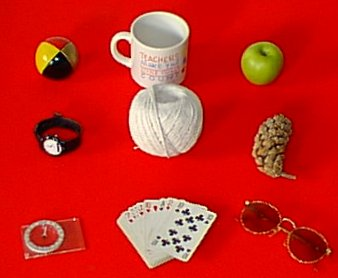While the magician isn't looking, the students are asked to choose an object an concentrate on it. The magician can quickly identify the chosen object by feeling the thought vibrations coming from it ... well not really. One of the students has previously been asked to act as the "plant" giving the magician a secret sign. Students are asked to work out some secret signs which could correctly identify one of the nine objects.

One system could be that the plant and the magician agree to define each object in terms of its row and column (like a matrix). The plant uses the position of their head to indicate the row and arms to indicate the column.

Students are encouraged to invent similar (more complex) illusions.

(alternatively this trick could be performed using nine buckets placed upside down on the floor with an item being put under one while the magician isn't looking.)

"Black Magic" is a well known old party trick in which a person apparently mind reads to find an object previously selected by an audience. An accomplice helps by pointing to various objects asking "is this it?". The mind reader always says no till after the accomplice has pointed to a black object; the next object to be pointed out will be the chosen object.
Students could devise similar mind reading tricks using number codes of other systems.

## Numbered Cards

One person thinks of a number 1 - 20. They are asked which cards contain their number. The magician can work out the number by adding up the top left numbers on each of the selected cards.How does it work? The numbers in the top left of each card are powers of two and any number can be expressed as a combination of powers of two. this can be shown to be the basis of the binary system.

This trick could be described by a teacher without showing the students a set of cards. Students challenged to make their own set of cards and extend for numbers greater than 20 (the trick works better the more numbers there are on each card). Students should practice performing this trick on each other using their own set of cards

Here's a more detailed Interactive version
The Online Psychic

## Real Magic Square

Wouldn't it be impressive if you could instantly write down a 4x4 magic square giving any total that a friend suggests. It can be done ... there's a trick to it!

First you have to memorise the basic 4x4 magic square which gives a total of 34. (Memorising this could lead to some interesting multisensory work!)

 14 1 12 7 11 8 13 2 5 10 3 16 4 15 6 9

Let's suppose your friend chooses the number 57. Firstly subtract 33 from this giving 24.

Now replace the numbers 1, 2, 3 and 4 in the basic square with 24, 25, 26 and 27 in that order.

You now have a magic square with 57 as the sum of each column, each row, each diagonal and each 2 by 2 square within the larger square.

Why does this work? Look at the positions of the numbers 1 - 4. How are they significant?

## Three Consecutive Primes (7,11,13)

The effect is that a pupil comes up with a three digit number and you challenge them to perform a calculation on that number using a calculator. You are always able to find the answer faster than thay can work it out.

The calculation involves multiplying the three digit number by 7, then the product is multiplied by 11 and then 13.

While the pupil is busy pressing buttons on the calculator you write down the three digit number twice to produce a six digit number which is the correct answer.

Example: 619

619 x 7 x 11 x 13

= 619619

It works because 7x11x13 is 1001.

## Odds and Evens

Ask a friend to hold an odd number of coins in one hand and an even number of coins in the other but not to let you see. You will be able to work out in which hand the odd number of coins is hidden.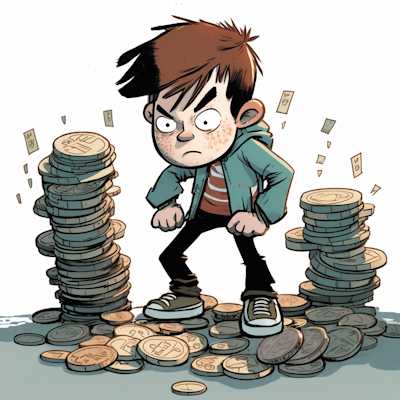Ask the friend to multiply the number of coins in their left hand by three and the number of coins in their right hand by four then add the two totals together.

If the sum of their totals is odd then the odd number of coins is in their left hand otherwise the odd number of coins is in their right hand.

For a clue to realising why this trick works see Mix and Math.

Finally, a trick that is guaranteed to impress everyone you show it to. A trick people will beg you to show them how it works. This trick is truly mathemagical.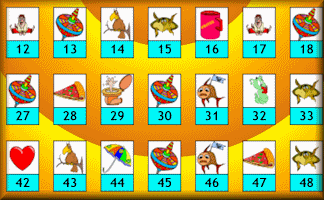As this is the last trick in our collection we will let you ponder over it and discover the method for yourself. It is simple mathematics and if you try it enough times, keeping a record of your results, the secret will become clear. Good luck!

## The Kruskal Count

D. Boyce, Rohwell

Monday, August 26, 2013

"This has saved me a lot of time over my summer holidays - thank you."

Saturday, October 19, 2013

"Thank you and blessings."

Sunday, September 6, 2015

"I did the collaborative choice 'trick' with yr7 last lesson Friday. Answer in an envelope. They whooped!"

Kyle D Evans, Maths Tricks To Blow Your Mind

Monday, May 30, 2022

"On your calculator, multiply together ten random single-digit numbers. You can use the same number more than once. You should now have a fairly long number. Cover the first digit and add up all the other digits. Now you either have a single-digit number or a two-digit number. If you have a single-digit number, move to the next step. If you have a two-digit number, add those digits to make a single-digit number, then move to the next step. Subtract your number from 9. Now uncover the digit you’ve been covering. Ta-dah!"

Do you have any thoughts about the mathemagic above? Do you know any mathemagical tricks that we could share with the world on this page? Click on the link below to submit your ideas.